# DAV Class 7 Maths Chapter 8 Worksheet 4 Solutions

The DAV Maths Book Class 7 Solutions Pdf and DAV Class 7 Maths Chapter 8 Worksheet 4 Solutions of Triangle and Its Properties offer comprehensive answers to textbook questions.

## DAV Class 7 Maths Ch 8 WS 4 Solutions

Question 1.
The hypotenuse of a right triangle is 17 cm long. If one of the remaining two sides is of length 8 cm, find the length of the third side. A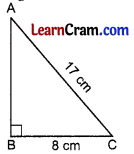In right ΔABC, ∠B = 90°
∴ From Pythagoras Theorem,
AB2 + BC2 = AC2
⇒ AB2 + (8)2 = (17)2
⇒ AB2 + 64 = 289
⇒ AB2 = 289 – 64 = 225
⇒ AB = 15cm
Hence the third side is 15 cm.

Question 2.
The length of the hypotenuse of a right triangle is 13 cm. If one of the side of the triangle be 5cm long, find the length of the other side.
In the figure given alongside,ABC is a right triangle in which
∠B = 90°
∴ From Pythagoras theorem, we get
AB2 + BC2 = AC2
⇒ AB2 + (5)2 = (13)2
⇒ AB2 + 25 = 169
⇒ AB2 = 169 – 25
⇒ AB2 = 144
AB = 12 cm
Hence the required side = 12 cmQuestion 3.
The length of two sides of a right triangle are equal. The square of hypotenuse is 800 cm2. Find the length of each side.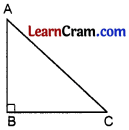In right triangle ABC, ∠B = 90°
∴ AC2 = 800
AB = AC (given)
∴ From Pythagoras theorem, we get
AB2 + BC2 = AC2
⇒ AB2 + AB2 = AC2
⇒ 2AB2 = AC2
⇒ 2AB2 = 800
⇒ AB2 = $$\frac{800}{2}$$ = 400
∴ AB = $$\sqrt{400}$$ = 20 cm
Hence AB = BC = 20 cm

Question 4.
Two poles of heights 6 m and 11 m stand on a plane ground. If the distance between their feet is 12 m, find the distance between their tops.
In the figure given alongside AB = 6m, CD = 11m and BD = 12m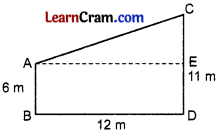Draw AE ∥ BD
CE = CD – ED
= CD – AB = 11m – 6m = 5m
and BD = AE = 12 m
From Pythagoras theorem, in right triangle AEC
AE2 + CE2 = AC2
⇒ (12)2 + (5)2 = AC2
⇒ 144 + 25 = AC2
⇒ 169 = AC2
∴ AC = $$\sqrt{169}$$ = 13 m
Hence the distance between their tops is 13 m.

Question 5.
Find the length of the diagonal of a rectangle whose sides are 15 cm and 8 cm.
Here is the given figure AB = 15 cm, BC = 8 cm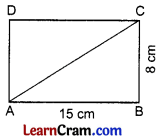In right triangle ABC,
AB2 + BC2 = AC2 (From Pythagoras theorem)
⇒ (15)2 + (8)2 = AC2
⇒ 225 + 64 = AC2
⇒ 289 = AC2
AC = $$\sqrt{289}$$ = 17 cm
Hence the length of the diagonal = 17 cm.Question 6.
The foot of a ladder is 6 m away from a wall and its top reaches a window 8m above the ground. If ladder is shifted in such a way that its foot is 8 m away from the wall, to what height does its top reach?
From Fig. (i),
AC2 = AB2 + BC2 (From Pythagoras theorem)
AC2 = (8)2 + (6)2
AC2 = 64 + 36 = 100
AC = $$\sqrt{100}$$ = 10 mNow in Fig. (ii),
DE = 10 m, EF = 8 m
∴ DE2 + EF2 = DF2 (from Pythagoras theorem)
⇒ DE2 + (8)2 = (10)2
⇒ DE2 + 64 = 100
⇒ DE2 = 100 – 64 = 36
∴ DE = $$\sqrt{36}$$ = 6 m
Hence the required height is 6 m.

Question 7.
A right angled triangle is isosceles. If the square of the hypotenuse is 50 m2, what is the length of each of its sides?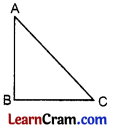From the given figure
AC2 = 50 m2
AB = BC
∴ From Pythagoras theorem, we get
AB2 + BC2 = AC2
⇒ AB2 + AB2 = 50
⇒ 2AB2 = 50
⇒ AB2 = $$\frac{50}{2}$$ = 25
∴ AB = $$\sqrt{25}$$ = 5 m
Hence AB = BC = 5m.

Question 8.
If the sides of a triangle are 3m, 4m and 6m long, determine whether the triangle is a right angled triangle.
Here, (6)2 = 36
(4)2 = 16 and (3)2 = 9
16 + 9 ≠ 36
Hence the given triangle is not a right triangle.Question 9.
Verify whether the following triplets are Pythagorean or not.
(i) (8,9,10)
8,9,10
(10)2 = 100, (9)2 = 81, (8)2 = 64
100 ≠ 81 + 64
100 ≠ 145
Hence it is not a Pythagorean triplet.

(ii) (5,7,12)
5, 7, 12
(12)2 = 144, (7)2 = 49, (5)2 = 25
144 ≠ 49 + 25
144 ≠ 74
Hence it is not a Pythagorean triplet.

(iii) (3,4,5)
3, 4, 5
(5)2 = 25, (4)2 = 16, (3)2 = 9
25 = 16 + 9
25 = 25
Hence it is a Pythagorean triplet.

(iv) (5,12,13)